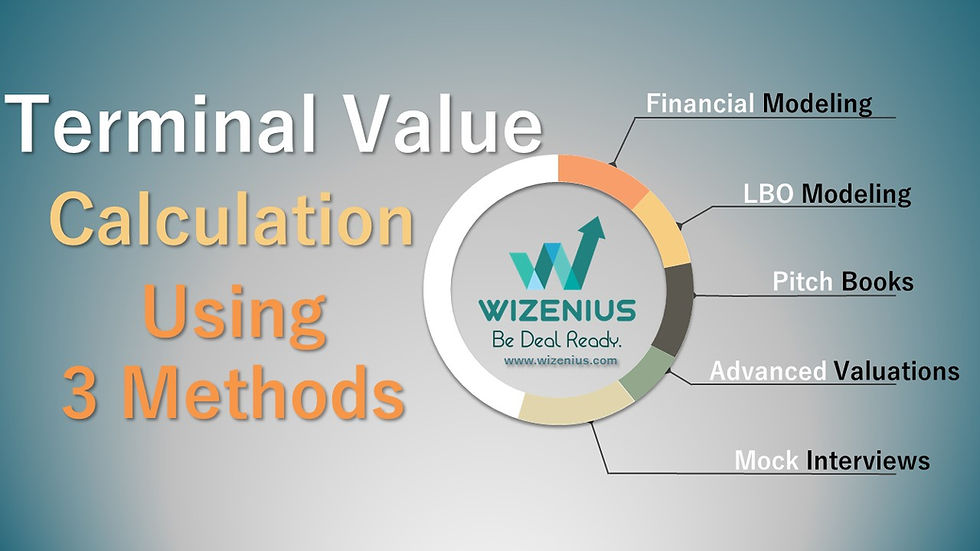top of page
Search

# Terminal Value Calculation using 3 Methods## Terminal Value

The terminal value is an essential component of a discounted cash flow (DCF) analysis. It represents the value of a business or an investment beyond the explicit projection period used in the DCF model. The terminal value captures the long-term cash flow generating potential of the company and accounts for the assumption that a business will continue to operate and generate cash flows beyond the forecasted period.

### Here are some reasons why the terminal value is important in a DCF analysis:

1. Capturing the long-term value: The DCF model typically forecasts cash flows over a specific projection period, which is usually around 5-10 years. However, most companies have a longer lifespan and continue to generate cash flows well beyond that period. The terminal value allows you to capture the value of these future cash flows and provide a more comprehensive assessment of the investment's worth.

2. Stable assumptions: Estimating cash flows over an extended period can be challenging due to uncertainties and changing market dynamics. The terminal value allows you to make more stable assumptions for cash flows beyond the explicit projection period. It simplifies the forecast by assuming a steady growth rate or stable cash flow multiples, which can be easier to estimate and rely on for long-term valuation.

3. Time value of money: The terminal value accounts for the time value of money by discounting future cash flows back to their present value. Since the terminal value represents cash flows further into the future, it is heavily discounted to reflect the reduced certainty and increased risk associated with distant cash flows. This ensures that the terminal value contributes a proportionate amount to the overall valuation.

4. Value creation potential: The terminal value captures the potential for value creation in the long term. It considers the expectation that a company's growth rate will stabilize and generate cash flows at a sustainable rate. This reflects the idea that mature companies may not experience the high growth rates seen in the initial years but can still generate significant value by maintaining stable operations.

5. Comparable valuation: The terminal value is also crucial for comparing different investment opportunities. By calculating the terminal value for multiple investments, you can assess their relative attractiveness. It provides a common ground for evaluating the long-term potential and allows for a standardized comparison of investment options.

## 3 Methods of Terminal Value Calculations

There are several methods commonly used to calculate the terminal value in a discounted cash flow (DCF) analysis. The choice of method depends on various factors, such as the nature of the business, industry dynamics, and the available data. Here are three widely used approaches:

1. Perpetuity Growth Method (Gordon Growth Model):

The perpetuity growth method assumes that the company's cash flows will grow at a stable rate indefinitely. This method is based on the Gordon Growth Model and calculates the terminal value using the following formula:

Terminal Value = Final Cash Flow × (1 + Growth Rate) / (Discount Rate - Growth Rate)

In this formula, the final cash flow represents the expected cash flow in the last year of the explicit forecast period, the growth rate is the assumed long-term growth rate, and the discount rate is the rate used to discount the cash flows.

2. Exit Multiple Method:

The exit multiple method estimates the terminal value by applying a suitable multiple to a financial metric such as earnings, EBITDA (earnings before interest, taxes, depreciation, and amortization), or free cash flow. The multiple is typically derived from comparable companies or transactions in the industry. The formula for the terminal value using the exit multiple method is:

Terminal Value = Final Year Financial Metric × Exit Multiple

Here, the final year financial metric represents the expected financial metric in the last year of the explicit forecast period, and the exit multiple is the selected multiple applied to that metric.

3. Return on Invested Capital (ROIC) or Return on Equity (ROE) Method:

The ROIC or ROE method calculates the terminal value based on the assumption that the company will generate a stable return on invested capital or equity in perpetuity. This method is particularly useful for capital-intensive industries. The formula for the terminal value using the ROIC or ROE method is:

Terminal Value = Final Year Invested Capital or Equity × Stable ROIC or ROE

Here, the final year invested capital or equity represents the expected invested capital or equity in the last year of the explicit forecast period, and the stable ROIC or ROE is the assumed long-term return rate.

It's important to note that the choice of the method and the specific inputs used to calculate the terminal value require careful consideration and should be based on sound judgment and analysis. Additionally, sensitivity analysis should be performed to assess the impact of changes in the terminal value assumptions on the overall valuation.